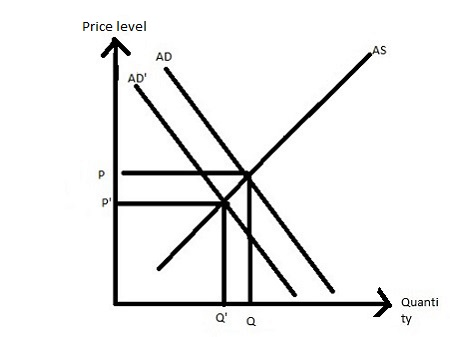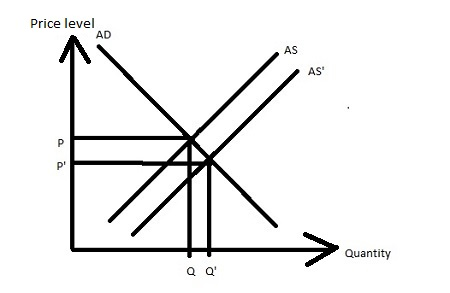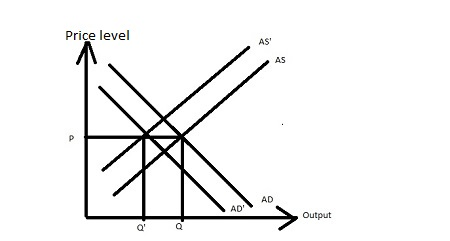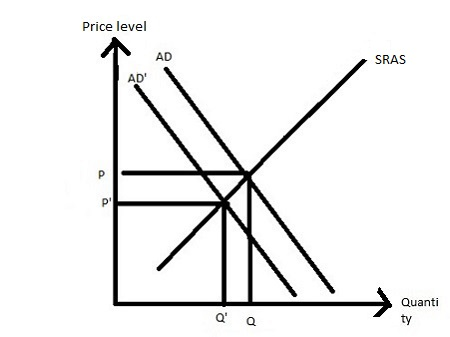# Illustrate each of the following situations with a graph showing the AS and AD curves, and...

## Question:

Illustrate each of the following situations with a graph showing the AS and AD curves, and explain what happens to the equilibrium values of the price level and aggregate output.

a) A decrease in G with the money supply held constant by the Fed.

b) A decrease in the, price of oil with no change in G.

c) An increase in the price of oil and a decrease in G.

d) An increase in the exchange rate (e.g., the number of Japanese Yen per US dollar increases).

## Aggregate demand and Aggregate supply:

The aggregate demand curve is the curve which shows the negative relationship between the price level and quantity demanded of real GDP. The aggregate demand curve is downward sloping.

The aggregate supply curve is the curve which shows the positive relationship between the price level and quantity supplied of real GDP. The aggregate supply curve is upward sloping.

## Answer and Explanation:

a) As the government expenditure decreases with the same money supply curve, then the aggregate demand curve shifts leftward, which reduce both price level and output.b) As the price of oil decreases with the same government expenditure, then the aggregate supply curve shifts rightward, which reduce the price level and increases the quantity.c) An increase in the price of oil shifts the aggregate supply curve leftward and at the same time, the government expenditure decreases, which results in a decrease in the aggregate demand. As a result, the output decreases, and the price level remains the same, assuming that the decline in aggregate supply is identical to a fall in aggregate demand.d) An increase in the exchange rate results in a decrease in exports, which results in the aggregate demand shifts leftward, which reduces both price level and output.#### Learn more about this topic:Aggregate Supply and Aggregate Demand (AS-AD) Model

from Economics 102: Macroeconomics

Chapter 7 / Lesson 3
58K